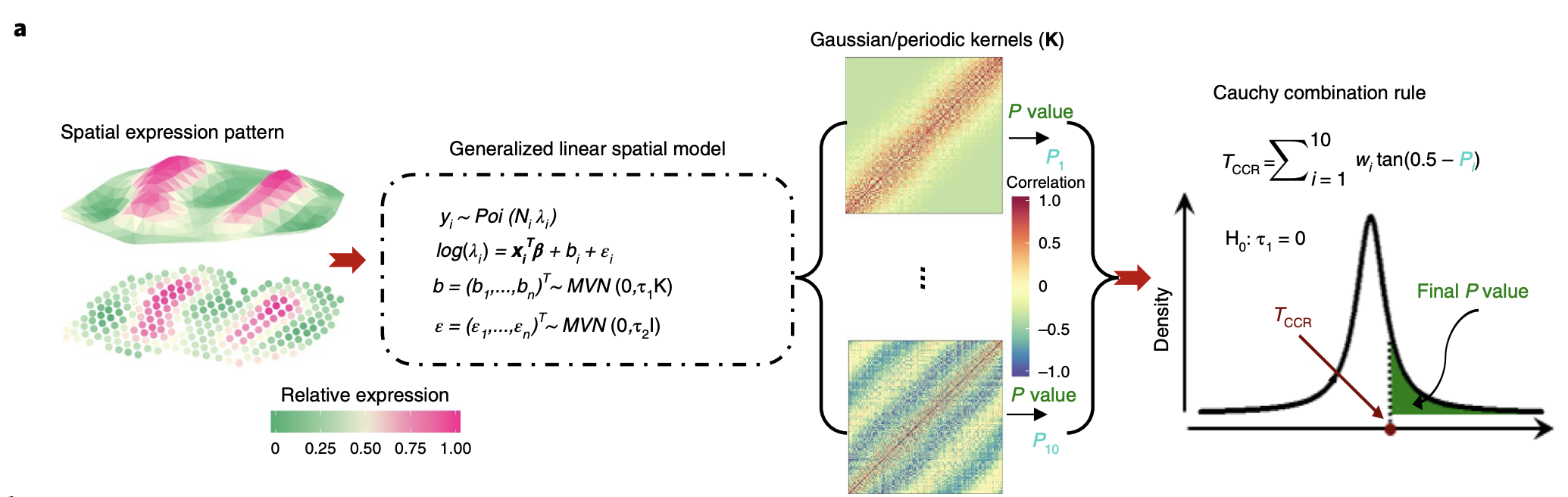# Sun et al. 2020 Nature Methods - Statistical analysis of spatial expression patterns for spatially resolved transcriptomic studies

Authors: Shiquan Sun, Jiaqiang Zhu, and Xiang Zhou

## Synopsis

This paper proposes a new method to determine whether given spatial gene expression data, there is any relationship between expression level and spatial coordinates. The method is built upon a generalized linear spatial model (GLSM) and  directly models count data unlike other methods (which transform count data before analysis), leading to better type 1 error control and higher power. It is also fully parametric and much more computationally efficient than non-parametric methods.

## Summary

### Figure 1AOverview of method. Spatial count data is modeled using generalized linear  spatial model (GLSM), where the spatial process is captured by a random effect. The GLSM has been previously used for interpolation and prediction of spatial data but is extended to hypothesis testing in the study. “Spatial coordinate” can be 3-D or include time as a dimension.

In the figure, $$y_i$$ represents the expression at a particular spatial coordinate for sample $$i$$, $$N_i$$ is a scale factor (used to normalize total expression of each coordinate across samples to be the same), and $$\lambda_i$$ is a Poisson rate parameter that depends on underlying gene expression level as well as sample $$i$$. $$\log(\lambda)$$ follows a linear model with $$x$$ representing a vector of covariates, $$\epsilon$$ representing residual error, and $$b$$ representing a random effect capturing spatial correlation patterns. $$b$$ for each spatial coordinate is drawn from a 0-mean multivariate normal with covariance $$K$$, which has a kernel function of the spatial locations.

The spatial kernel function can be specified arbitrarily in order to capture specific spatial patterns. For example, a Gaussian kernel is useful for clustered expression patterns, while a periodic kernel useful for oscillating expression pattern. The authors ended up picking 10 kernels (5 periodic kernels with different periodicity parameters and 5 Gaussian kernels with different smoothness parameters), then aggregate across all kernels.

The null hypothesis is that $$\tau_1 = 0$$. P-values were computed for each kernel using the Satterthwaite method, then aggregated across 10 kernels using the Cauchy combination rule.

*****
Written by Douglas Yao on 28 October 2020